SQL Server Calendar Table:  Tips and Tricks

Published on
8,963 Points
4,863 Views
6 Endorsements
A collection of tips and tricks when querying dates using a calendar table.
This is second in a series of articles on dealing with custom calendars in SQL Server, and requires that you execute the code at the bottom of the SQL Server Calendar Table article.

1. Customizable list of days
2. Boundaries of a range of dates, such as weeks, month, quarter, or year
3. A list of dates with an '< ALL >' choice.

Customizable list of days

This one is a handy way to pull off a list of days with a custom definition, such as 'The least seven days, starting with whatever day yesterday is', 'The seven days after 9/1/15', and 'The three days before 12/25/15, not including that day', and is a collection of many tricks I’ve picked up.

The below T-SQL code uses nested Common Table Expressions and these user-selectable parameters:

• @dt                                   The date passed.  Usually today.
• @previous_or_next        'P' all rows are before @dt, 'N' all rows after @dt.  Sorted from @dt going outwards.
• @include_dt                    'Y' include the @dt day/week/whatever in the return set, 'N' do not include.
• @row_count                    Number of rows in the return set.

The same code is demonstrated below as both a Stored Procedure and a Table-Valued Function
• Stored Procedure          Returns a set, Used by SSRS as a data source,
• Table Valued Function  Returns a set, can be JOINed to other tables or views using CROSS APPLY or OUTER APPLY.

Written as a Stored Procedure

IF EXISTS(SELECT name FROM sys.procedures WHERE name='cal_list')
DROP PROC cal_list
GO

CREATE PROC cal_list (
@dt date = NULL,                  -- Start Date.  Today if a value is not passed.
@include_dt char(1) = 'Y',        -- Y Include @dt in the return set, N do not include
@previous_or_next char(1) = 'P',  -- P Previous .. before @dt, N next .. after @dt
@row_count int = 100) AS

/*
2015-09-016  Jim Horn,  Original.  http://www.jimhorn.biz  All copies of this code must include this line.
*/

-- TESTING ONLY, comment out the below line in production
-- Declare @dt date = '20150905', @include_dt char(1) = 'Y', @previous_or_next char(1) = 'P', @row_count int = 12

-- If date was passed as NULL, use today
SET @dt = CASE WHEN @dt IS NULL THEN CAST(GETDATE() as date) ELSE @dt END

;WITH days_rows AS (
SELECT TOP(@row_count + 1) PKDate
FROM days
WHERE
(@previous_or_next = 'P' AND PKDate <= @dt) OR
(@previous_or_next = 'N' AND PKDate >= @dt)
ORDER BY
CASE @previous_or_next WHEN 'P' THEN PKDate END DESC,
CASE @previous_or_next WHEN 'N' THEN PKDate END),
days_ranked AS (
SELECT PKDate, RANK() OVER (ORDER BY PKDate) as row_number
FROM days_rows),
days_ranked_max AS (SELECT MAX(row_number) as row_number_max FROM days_ranked)
SELECT PKDate
FROM days_ranked dr
WHERE (
(@previous_or_next = 'P' AND @include_dt = 'Y' AND row_number >= (SELECT row_number_max FROM days_ranked_max) - @row_count + 1) OR
(@previous_or_next = 'P' AND @include_dt = 'N' AND row_number <> (SELECT row_number_max FROM days_ranked_max) AND row_number >= (SELECT row_number_max FROM days_ranked_max) - @row_count) OR
(@previous_or_next = 'N' AND @include_dt = 'Y' AND row_number <= @row_count) OR
(@previous_or_next = 'N' AND @include_dt = 'N' AND row_number <> 1 AND row_number <= (@row_count + 1))
)
ORDER BY
CASE @previous_or_next WHEN 'P' THEN PKDate END DESC,
CASE @previous_or_next WHEN 'N' THEN PKDate END
GO
The results...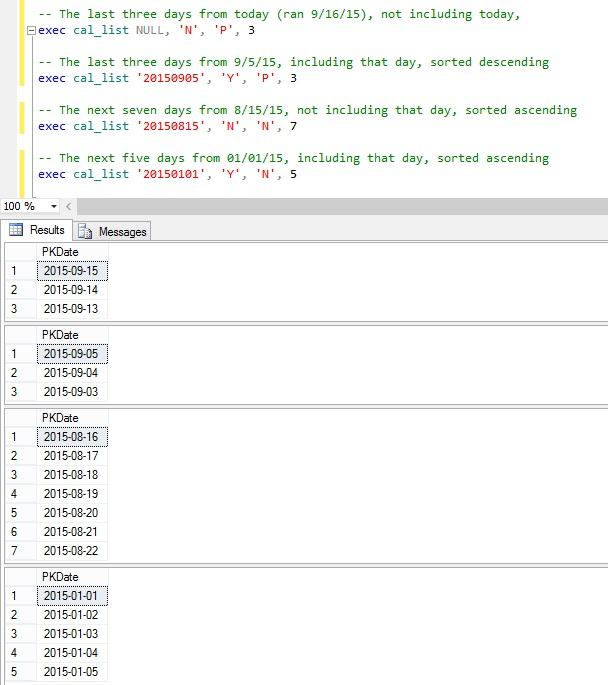Written as a Function

IF EXISTS(SELECT name FROM sys.objects WHERE name='fn_cal_list')
DROP FUNCTION fn_cal_list
GO

CREATE FUNCTION fn_cal_list(
@dt date = NULL,                  -- Start Date.  Today if a value is not passed.
@include_dt char(1) = 'Y',        -- Y Include @dt in the return set, N do not include
@previous_or_next char(1) = 'P',  -- P Previous .. before @dt, N next .. after @dt
@row_count int = 100)
RETURNS @ret TABLE (PKDate date)
AS

BEGIN
/*
2015-09-05  Jim Horn,  Original.  http://www.jimhorn.biz  All copies of this code must include this line.
*/

-- TESTING ONLY, comment out the below line in production
-- Declare @dt date = '20150905', @include_dt char(1) = 'Y', @previous_or_next char(1) = 'P', @row_count int = 12

-- If date was passed as NULL, use today
SET @dt = CASE WHEN @dt IS NULL THEN CAST(GETDATE() as date) ELSE @dt END

;WITH days_rows AS (
SELECT TOP(@row_count + 1) PKDate
FROM days
WHERE
(@previous_or_next = 'P' AND PKDate <= @dt) OR
(@previous_or_next = 'N' AND PKDate >= @dt)
ORDER BY
CASE @previous_or_next WHEN 'P' THEN PKDate END DESC,
CASE @previous_or_next WHEN 'N' THEN PKDate END),
days_ranked AS (
SELECT PKDate, RANK() OVER (ORDER BY PKDate) as row_number
FROM days_rows),
days_ranked_max AS (SELECT MAX(row_number) as row_number_max FROM days_ranked)
INSERT INTO @ret (PKDate)
SELECT PKDate
FROM days_ranked dr
WHERE (
(@previous_or_next = 'P' AND @include_dt = 'Y' AND row_number >= (SELECT row_number_max FROM days_ranked_max) - @row_count + 1) OR
(@previous_or_next = 'P' AND @include_dt = 'N' AND row_number <> (SELECT row_number_max FROM days_ranked_max) AND row_number >= (SELECT row_number_max FROM days_ranked_max) - @row_count) OR
(@previous_or_next = 'N' AND @include_dt = 'Y' AND row_number <= @row_count) OR
(@previous_or_next = 'N' AND @include_dt = 'N' AND row_number <> 1 AND row_number <= (@row_count + 1))
)
ORDER BY
CASE @previous_or_next WHEN 'P' THEN PKDate END DESC,
CASE @previous_or_next WHEN 'N' THEN PKDate END

RETURN
END

The results..

Boundaries of a range of dates, such as weeks, month, quarter, or year

This one is relatively easy with a calendar table, as all you have to do is query the appropriate column for the min and max date.   The below Stored Procedure performs this, and also includes parameters for the range type (week, month, quarter, year) and an offset value which will take the @dt and return the @interval range plus or minus @offset.

Most of the functionality is done with a CASE block in the WHERE clause, which combines the @interval value with an expression that uses the appropriate column.

• @dt                                   The date passed.  Usually today.
• @interval                         'Y' Year, 'Q' Quarter, 'M' Month, 'W' Week.
• @offset                            Positive number = Use @dt plus @offset number of @interval's, then get the range.  Negative number - minus.

IF EXISTS(SELECT name FROM sys.procedures WHERE name='cal_boundaries')
DROP PROC cal_boundaries
GO

CREATE PROC cal_boundaries (@dt date, @interval char(1) = 'M', @offset int = 0) AS

/*
Get the day boundaries of a given week that contains @dt, plus or minus @offset weeks.

2015-09-05  Jim Horn,  Original.  http://www.jimhorn.biz  All copies of this code must include this line.
*/

-- TESTING ONLY, comment out the below line in production
-- Declare @dt date = '20150905', @interval char(1) = 'M', @offset int = 0

SELECT MIN(PKDate) as dtBegin, MAX(PKDate) as dtEnd
FROM days
WHERE
(@interval = 'Y' AND continuous_year = (SELECT continuous_year FROM days WHERE PKDate = DATEADD(year, @offset, @dt))) OR
(@interval = 'Q' AND continuous_quarter = (SELECT continuous_quarter FROM days WHERE PKDate = DATEADD(quarter, @offset, @dt))) OR
(@interval = 'M' AND continuous_month = (SELECT continuous_month FROM days WHERE PKDate = DATEADD(month, @offset, @dt))) OR
(@interval = 'W' AND continuous_week = (SELECT continuous_week FROM days WHERE PKDate = DATEADD(week, @offset, @dt)))
GO
The results..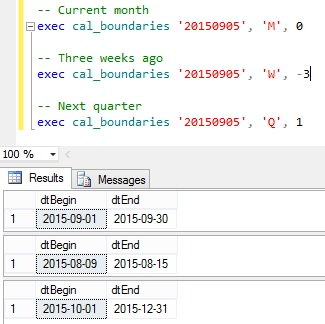A list of dates with an < ALL > choice

Here’s what you need to pull this off:
• Add a UNION ALL to your original query to include a row for the < ALL > and a value of -1.
• Ensure correct sorting by verifying if the -1 is the first row in the number column, and if not use a separate column sort_order.
• Then in whatever query uses this selection handle -1 as all rows.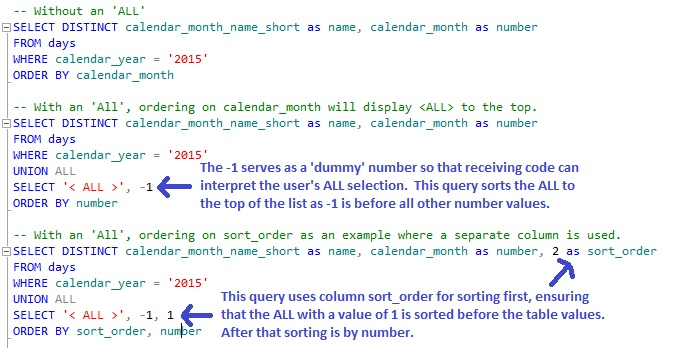The results.  The first query produces the set on the left, and the second and third query will produce the set on the right.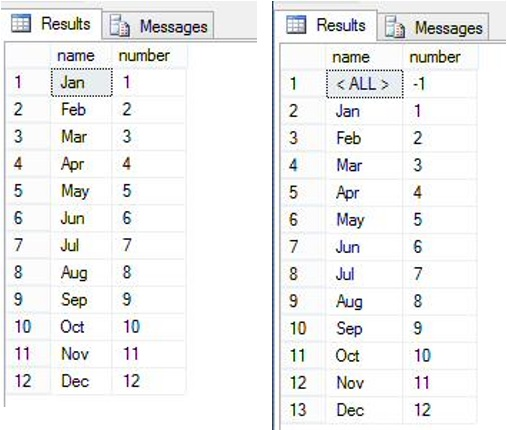Then the Stored Procedure that accepts the @month parameter goes something like this: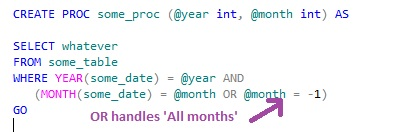Thank you for reading my article, feel free to leave me some feedback regarding the content or to recommend future work.  If you liked this article please click the 'Good Article' button.

I look forward to hearing from you. -  Jim - ( LinkedIn ) ( Twitter )

6